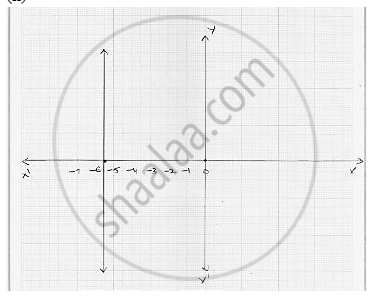# Give the Geometrical Representation of 2x + 13 = 0 as an Equation In Two Variables . - Mathematics

Give the geometrical representation of 2x + 13 = 0 as an equation in two variables .

#### SolutionTwo variable representation of  2x + 13 = 0

2x + 0y + 13 = 0

2x + 13 = 0

2x = - 13

 x = (- 13)/ 2

x = - 6 . 5

On Cartesian plane, equation represents all points  y - axis  for which x = - 6 . 5 .

Concept: Equations of Lines Parallel to the X-axis and Y-axis
Is there an error in this question or solution?

#### APPEARS IN

RD Sharma Mathematics for Class 9
Chapter 7 Linear Equations in Two Variables
Exercise 7.4 | Q 2.2

Share